# Numeracy Goal

All students will demonstrate number sense and will meet essential learning goals in numeracy at each grade level by June.

Number sense’ refers to the ability to understand the value of a number and represent the number in different ways.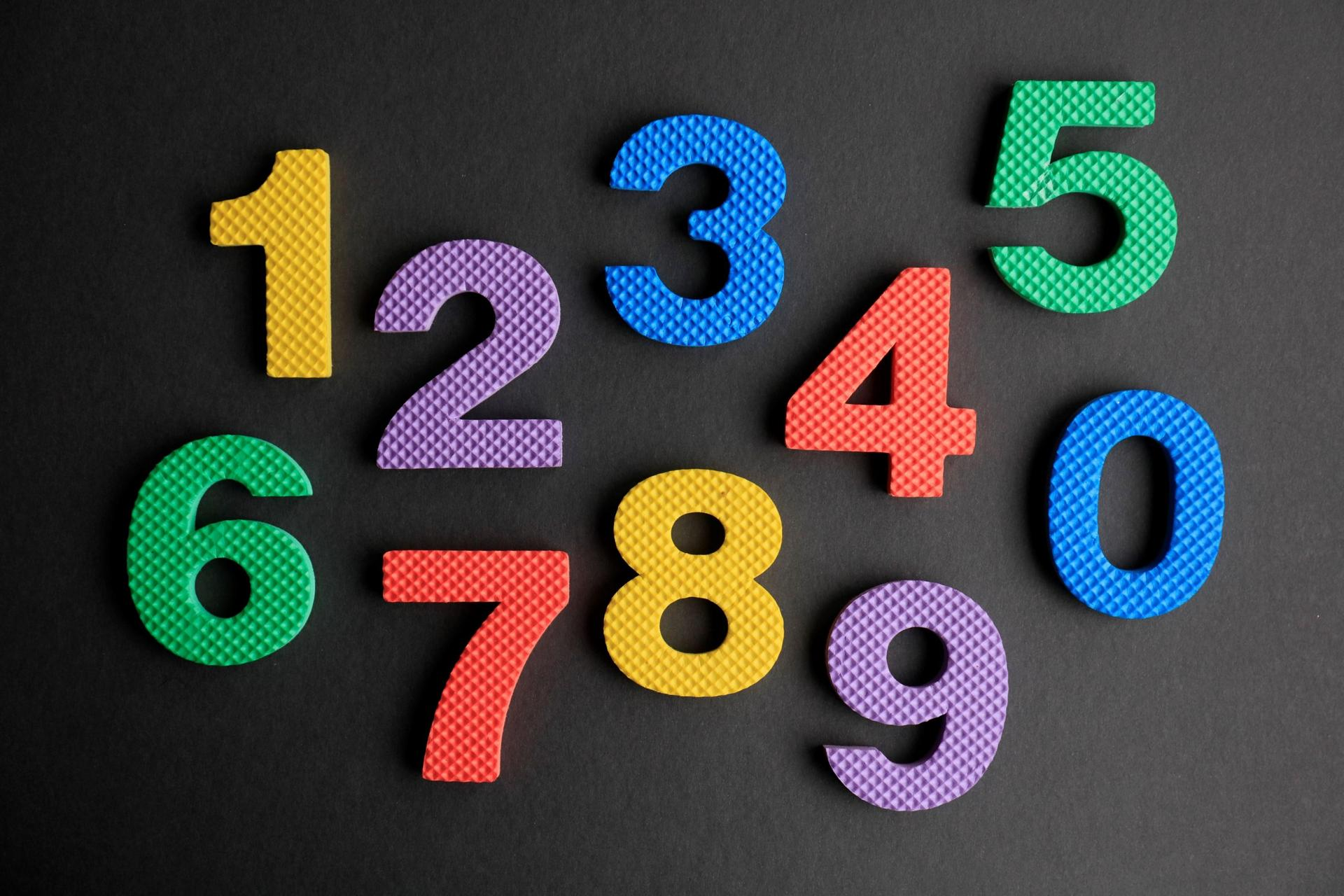Ways We Will Support This Goal

Revisit essential learning outcomes to confirm common goals and language across grades. Revisiting curricular content continues to be important to address missed learning time due to the pandemic.
Daily calendar routines/ High Yield routines/ use of 100's charts
Counting by 5,10, 1-20 (forwards,backwards) and counting songs, incorporating movement
Partner work, math centers and math games
Use of SNAP math tool to improve number sense strategies
Use of Tara West Math resources and Jump Math resources to support instruction.
Use of math manipulatives to support visual representations
Mathletics integrated into schedules for grades 1-3
Assess often and intervene when needed using RTI model.
Student support within the classroom by Learning Support Workers.
How Will We Know?

Use of the SNAP Math tool (attached below) as both a formative and summative assessment tool for grades 1-3.

Assessment of grade 3 students will include data from the District Numeracy Assessment performed in Fall and Spring which focuses specifically on number sense and problem-solving.

Numeracy Data

This year, the district is using two new assessments to focus on number sense and problem solving for grade three students.

The SNAP Numeracy assessment is a tool to measure number sense. ‘Number sense’ refers to the ability to understand the value of a number and represent the number in different ways (using real-life examples, representing using math tiles, on a number line, etc). We know that strong number sense supports students’ achievement in all areas of math. In addition to other strategies to support numeracy, we continue to use SNAP Math tool as both a practice tool and an assessment tool.

The problem solving assessment gives students a choice of two problems; students choose one problem to analyze, solve and explain their reasoning. Problem Solving assessment data was collected once this year in January 2022.

The 2021-22 data for grade three shows that most students were either developing or showing proficiency in number sense and problem-solving skills. A focus area for instruction moving forwards is to support students to reflect on their own mathematical thinking and connecting concepts to real-life (connecting and reflecting).  The June number sense (SNAP) data showed growth in connecting and reflecting.

June 2022 NUMBER SENSE (SNAP) Assessment – grade 3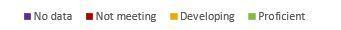Communicating & Representing Can draw a picture to represent a number and explain it. Can expand a number.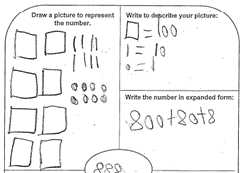FALL 2021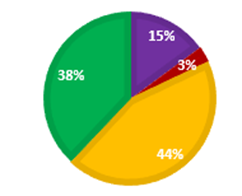JUNE 2022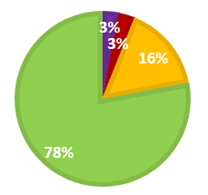By the end of the year, 78% of students were proficient in this area. Understanding & Problem-solving Can create math equations to show understanding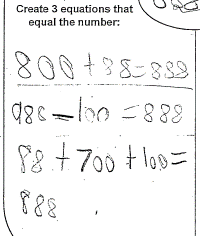FALL 2021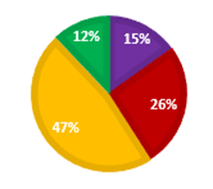JUNE 2022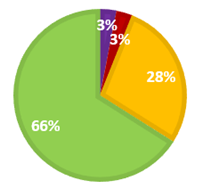By the end of the year, 66% of students were proficient in this area. Connecting & Reflecting Can give real-life examples of a number   There are 888 students at a large high school. There are four grades and over 200 students in each grade. FALL 2021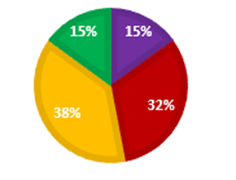JUNE 2022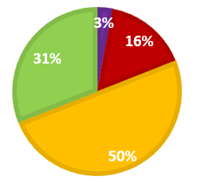By the end of the year, 31% of students were proficient in this area. Reasoning & Analyzing Can count above and below the number.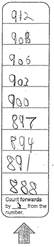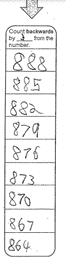Can show where the number would fit on a number line.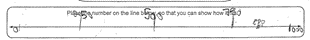FALL 2021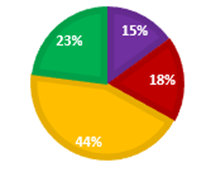JUNE 2022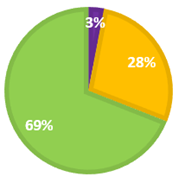By the end of the year, 69% of students were proficient in this area.

January 2022 PROBLEM SOLVING Assessment – grade 3

 PROBLEM SOLVING SKILL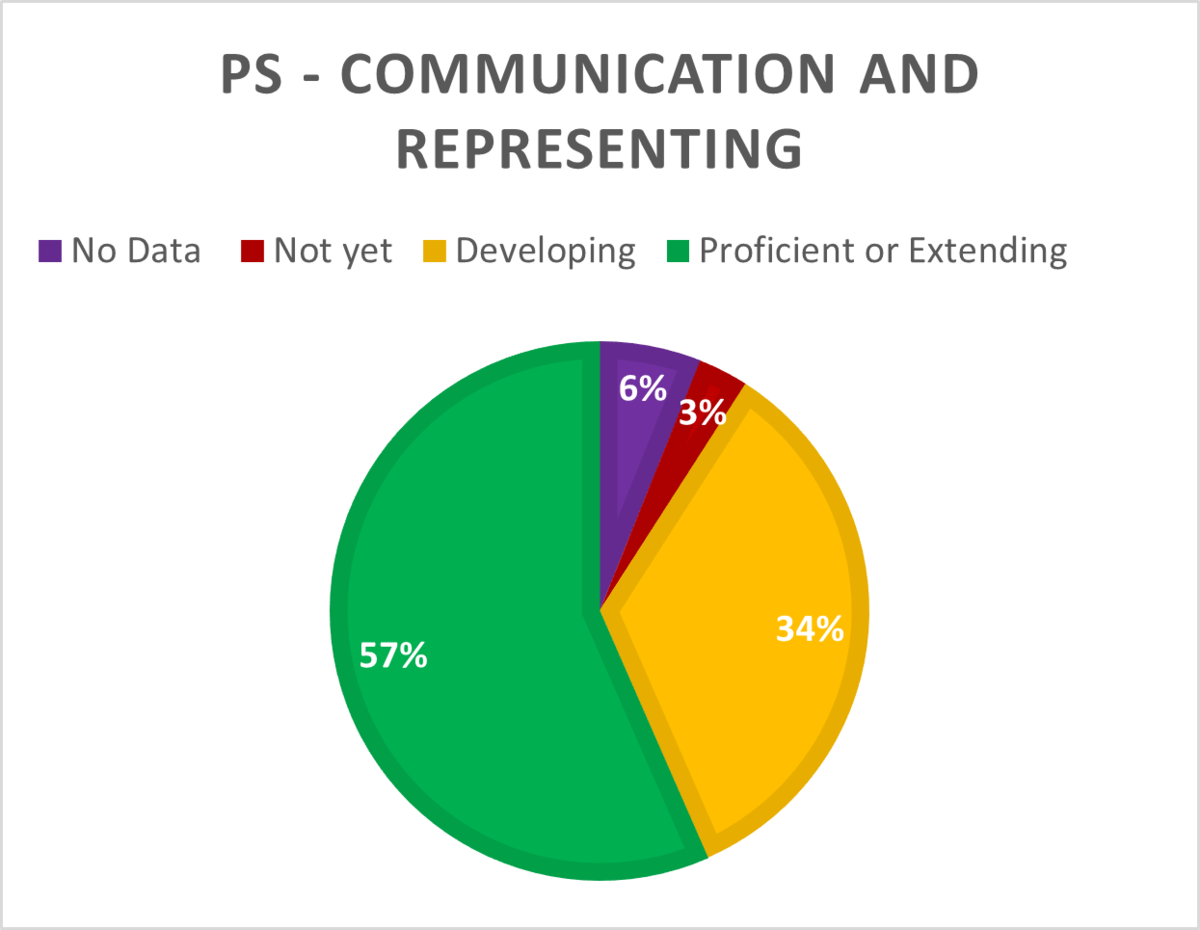Can explain solution uses mathematic language/ units represents the problem uses organizers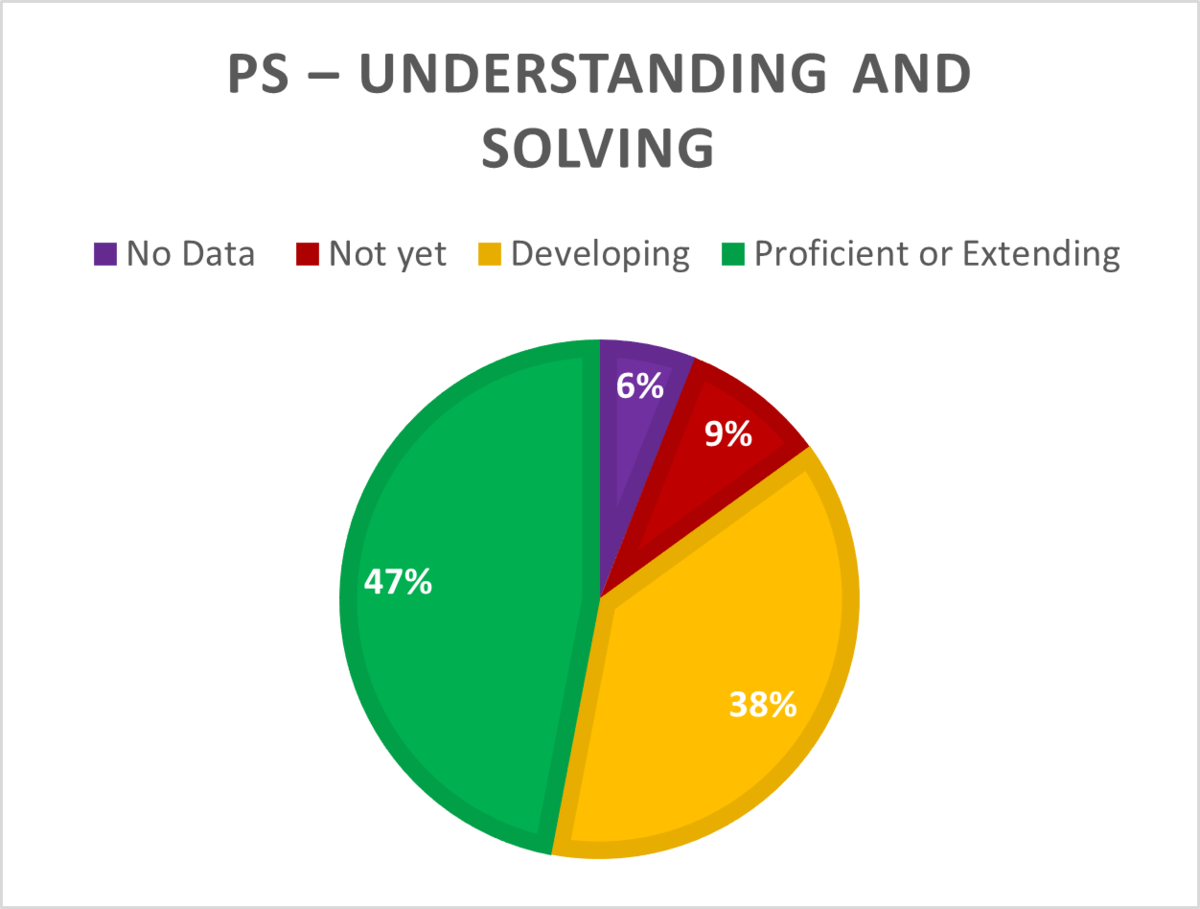Chooses appropriate strategy and presents a solution that makes sense uses math concepts and skills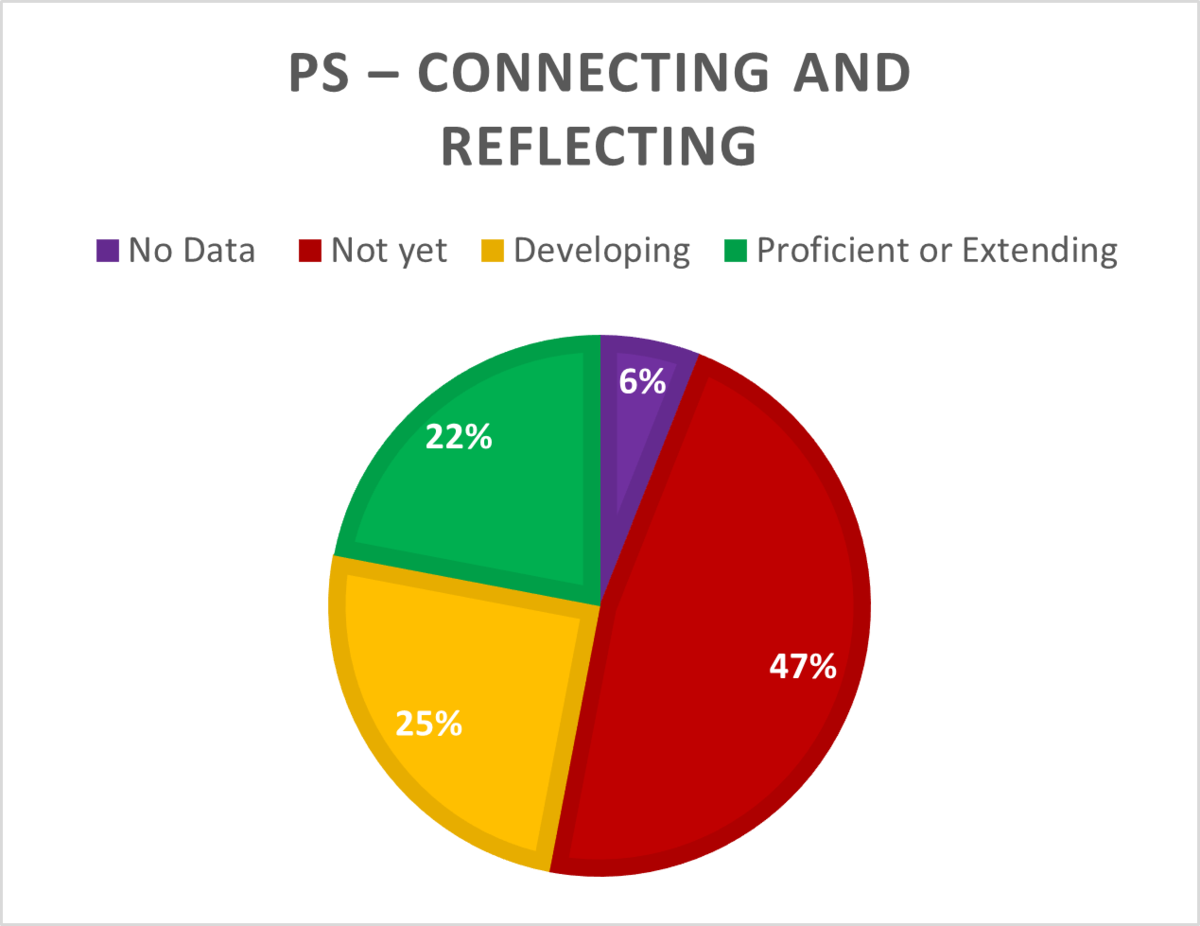Can reflect on own mathematical thinking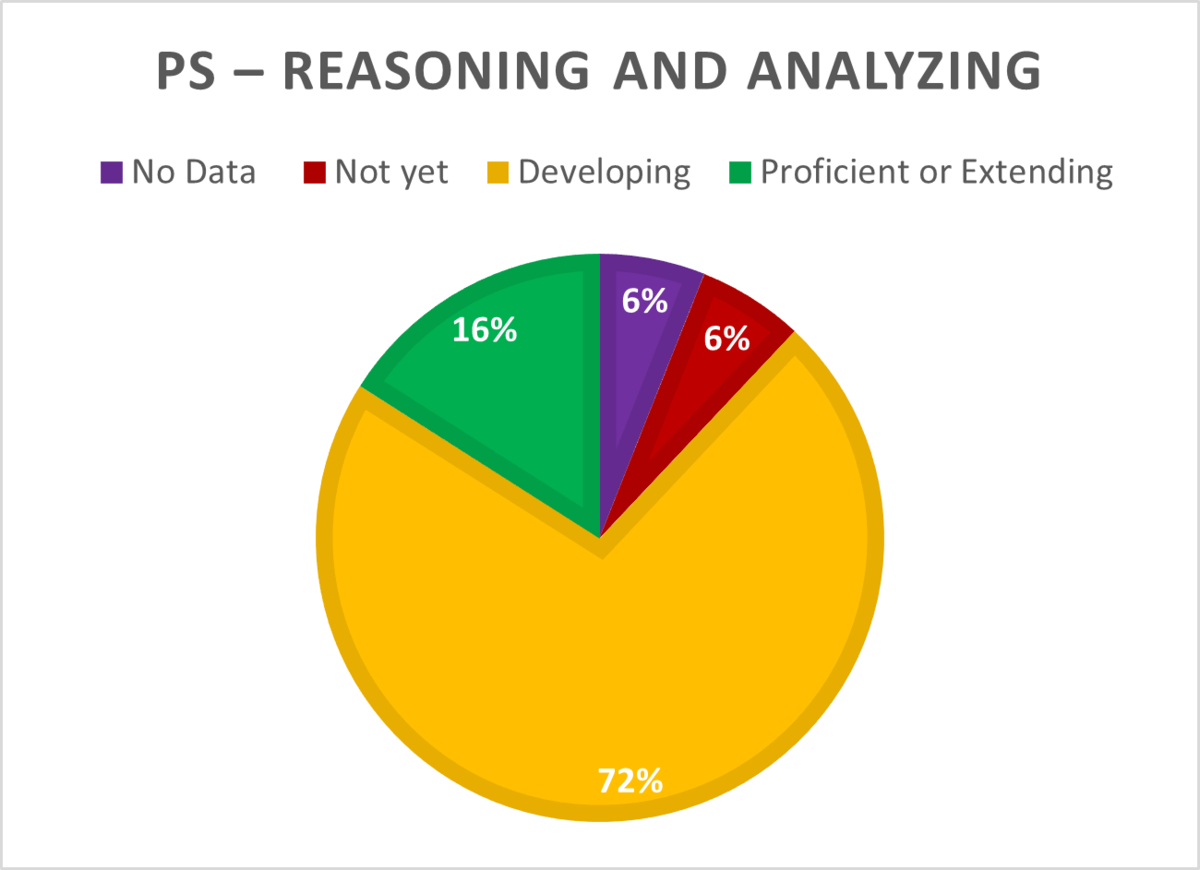Can explain reasoning shows work identifies constraints of solution
In prior years, numeracy data at Mouse Mountain has pointed to the development of number sense skills by grade 3. Last year's data at the end of grade 3 mirrored previous (pre-Covid) data. Given that our grade 3 students started the year with only 36% meeting expectations and ended the year with 80% meeting expectations, we observed that students made significant gains in their learning when instruction was not interrupted. As well, the data supports a strong numeracy program at the school.

Number Sense Data for Grade 3 Students in 2020-2021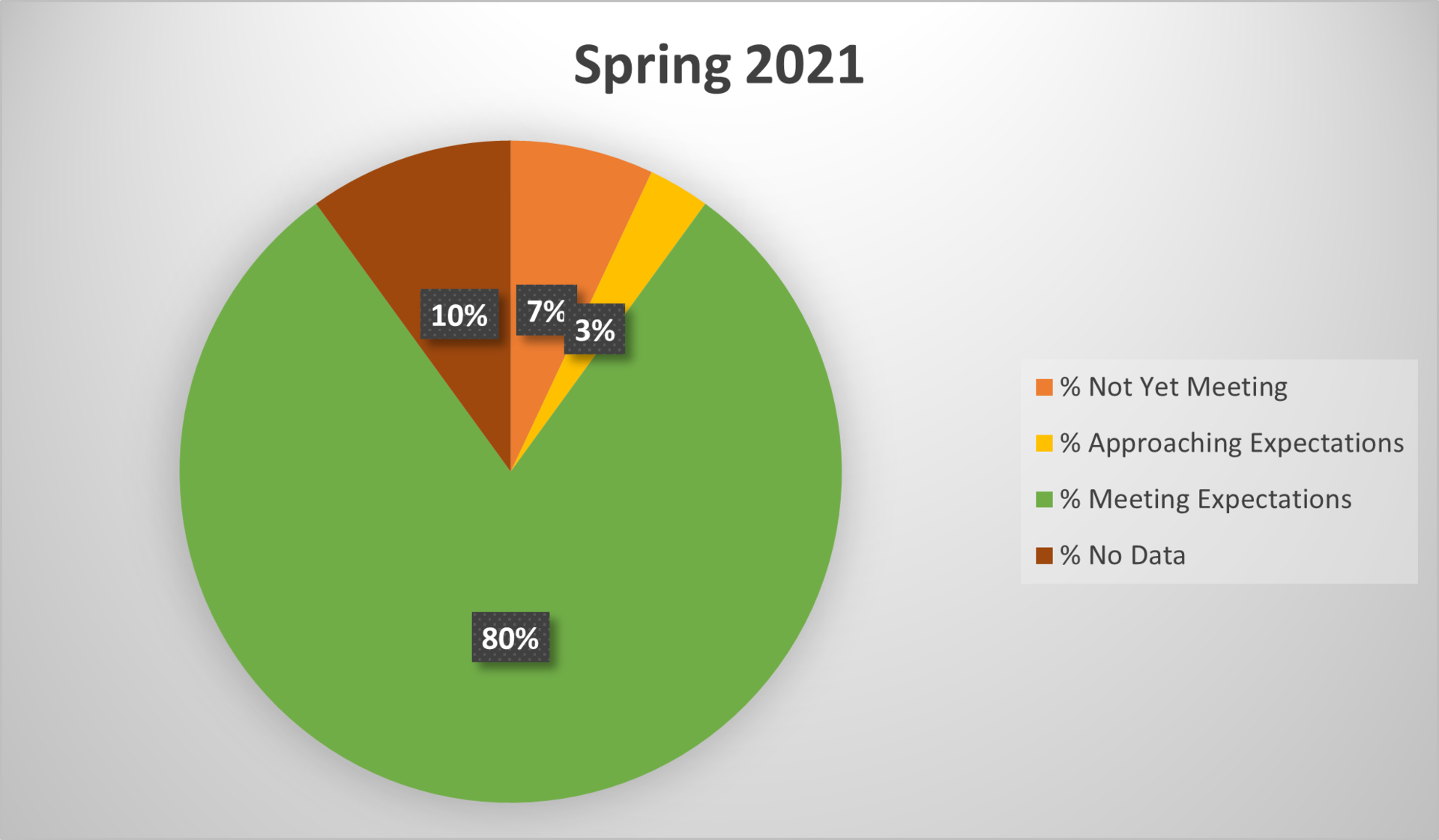80% of students were meeting expectations at the end of grade 3.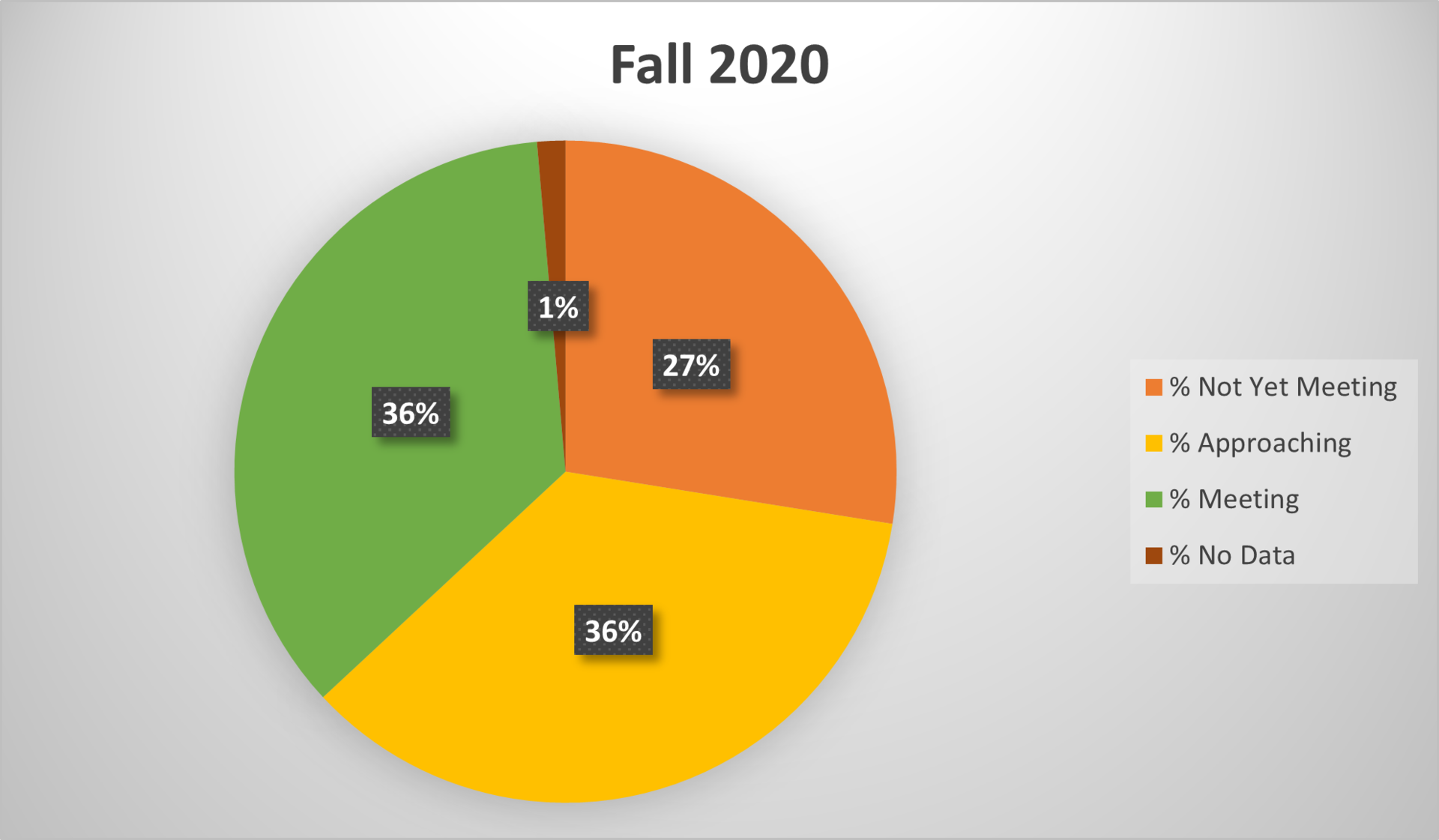36% of students were meeting expectations in the Fall of 2020 (beginning of grade 3, based on end of grade 2 expectations).